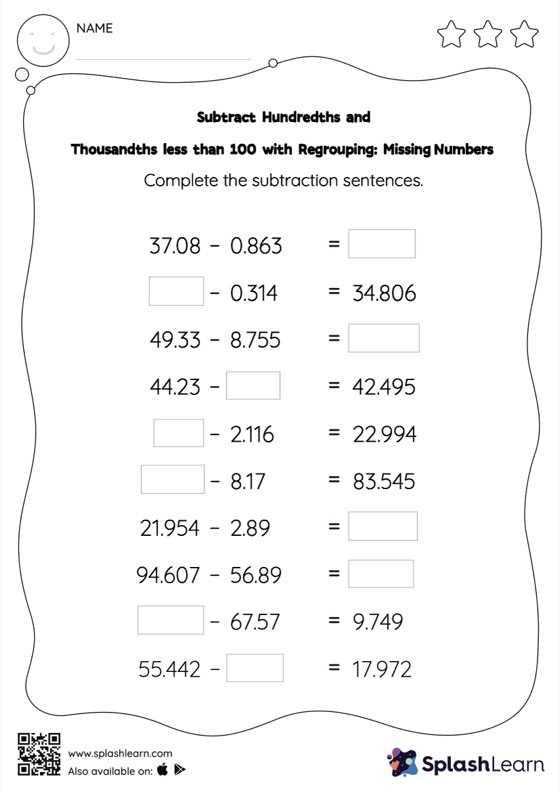# Subtract Hundredths and Thousandths less than 100 with Regrouping: Missing Numbers Worksheet

Home > Subtract Hundredths and Thousandths less than 100 with Regrouping: Missing NumbersThis worksheet challenges young mathematicians to hone their math skills by solving a set of problems on subtracting hundredths and thousandths less than 100 with regrouping. While subtracting decimals, students use the relationship between addition and subtraction to find the missing number. They also need to regroup numbers in subtract hundredths and thousandths less than 100 with regrouping worksheet utilizing the relationship between ones, tenth, hundredth, thousandth, etc., To get to the result.